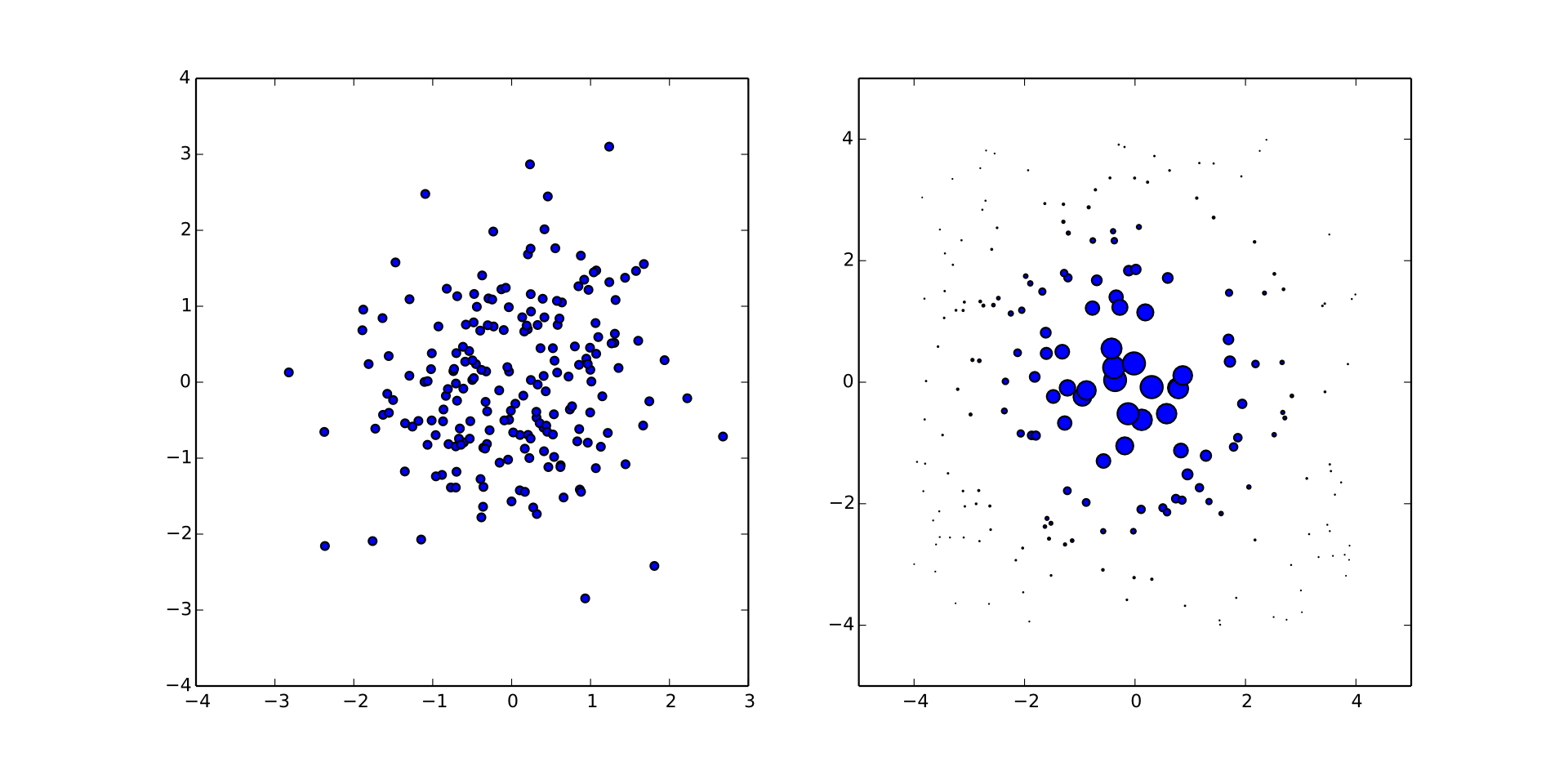# Quantum Bootstrapping

joint work with [Nathan Wiebe](http://research.microsoft.com/en-us/people/nawiebe/), Christopher Ferrie, and D. G. Cory [doi:10/tf3](https://dx.doi.org/10/tf3), [doi:10/tdk](https://dx.doi.org/10/tdk), and [doi:10/7nx](https://dx.doi.org/10/7nx) https://www.cgranade.com/research/talks/ipdf/10-2015/ $\newcommand{\ee}{\mathrm{e}} \newcommand{\ii}{\mathrm{i}} \newcommand{\dd}{\mathrm{d}} \newcommand{\id}{𝟙} \newcommand{\TT}{\mathrm{T}} \newcommand{\defeq}{\mathrel{:=}} \newcommand{\Tr}{\operatorname{Tr}} \newcommand{\Var}{\operatorname{Var}} \newcommand{\Cov}{\operatorname{Cov}} \newcommand{\rank}{\operatorname{rank}} \newcommand{\expect}{\mathbb{E}} \newcommand{\sket}{|#1\rangle\negthinspace\rangle} \newcommand{\sbraket}{\langle\negthinspace\langle#1\rangle\negthinspace\rangle} \newcommand{\Gini}{\operatorname{Ginibre}} \newcommand{\supp}{\operatorname{supp}} \newcommand{\CC}{\mathbb{C}}$

Citations are listed by handle (e.g. 10/abc or 10012/abc) or arXiv number.
handle $\mapsto$ https://dx.doi.org/handle

We want to build a quantum computer.

As a prerequiste, we must be able to characterize the dynamics of large quantum systems, and to calibrate our control for these dynamics.

Formally, we need to learn the Hamiltonian $H$ of a system.

### Our Strategy

- Express Hamiltonian learning in terms of simulation. - Substitute in quantum simulators.

Yields semiquantum algorithm that avoid classically-infeasible simulation calls.

We use physical insight to reduce the size of the quantum simulator needed.

Information locality provides a potentially-scalable characterization and calibration algorithm.

## Bayesian Inference

Allows us to reduce learning to simulation.

$$\Pr(\text{hypothesis} | \text{data}) = \frac{\Pr(\text{data} | \text{hypothesis})}{\Pr(\text{data})} \Pr(\text{hypothesis})$$

- Prior $\Pr(\text{hypothesis})$ describes initial knowledge. - Posterior $\Pr(\text{hypothesis} | \text{data})$ describes what we learn from $\text{data}$. - Likelihood $\Pr(\text{data} | \text{hypothesis})$ represents simulation of experimental system.

### Representing Hypotheses

We represent hypothetical Hamiltonians by a model $H = H(\vec{x})$ w/ params $\vec{x}$.

Example (Ising model): $H(\vec{x}) = \sum_{i,j} x_{i,j} \sigma_z^{(i)} \sigma_z^{(j)}.$

$\mathrm{O}(n^2)$ parameters vs $\mathrm{O}(2^{2n})$ parameters.

### Sequential Monte Carlo

Represents distributions by finite sums for efficient numerical implementation.

\begin{align} \Pr(\vec{x}) & = \sum_{p \in \text{particles}} w_p \delta(\vec{x} - \vec{x}_p) \\ w_p & \mapsto w_p \times \Pr(\text{data} | \vec{x}_p) / \mathcal{N} \end{align}

Simulation is a resource to perform Bayes updates.

Liu-West Resampling (10/8c2) dynamically moves samples to recover numerical stability.$\Pr(1 | \omega_1, \omega_2; t_1, t_2) = \cos^2(\omega_1 t_1 / 2) \cos^2(\omega_2 t_2 / 2)$

- Classical Hamiltonian learning: [Granade *et al.* 10/s87](https://dx.doi.org/10/s87), [Stenberg *et al.* 10/7nw](https://dx.doi.org/10/7nw) - Randomized benchmarking: [Granade *et al.* 10/zmz](https://dx.doi.org/10/zmz) - Tomography: [Huszár and Houlsby 10/s86](https://dx.doi.org/10/s86), [Ferrie 10/7nt](https://dx.doi.org/10/7nt), [Granade *et al.* 1509.03770](https://scirate.com/arxiv/1509.03770)

### QInfer

Extensible and flexible open-source library for Python.

### Easy to Use


import qinfer as qi
model = qi.BinomialModel(qi.SimplePrecessionModel())
prior = qi.UniformDistribution([[0, 1]])
updater = qi.smc.SMCUpdater(model, 1200, prior)


Enough classical, though, let's get back to

### Quantum Hamiltonian Learning.

Replace likelihood calls in the Bayes update with quantum likelihood evaluation, using trusted device.

Heuristics for experiment design are critical.

### Particle Guess Heuristic

• Draw $H_-$, $H_-'$ from posterior.
• Evolve under $H_-$ for $t = 1 / \|H_- - H_-'\|$.

Simulation-free, uses current uncertainty to choose next experiment s.t. $t \|H_- - H\| \approx \text{const}$.

### QHL is Robust

We obtain accurate results even with sampling error, imperfect coupling, and approximate models.

Since QHL is robust to approximate models, use information locality (Lieb-Robinson bounds) to provide approximate compressed model.

Scan observable across trusted register and untrusted device.

Perform multiple rounds to reduce Lieb-Robinson velocity by current knowledge of $H$.

50-qubit untrusted device, 8-qubit simulator, 4-qubit observable

### Calibrating for Crosstalk

Iterate compressed quantum Hamiltonian learning for each control Hamiltonian $H_i$.

$H(\vec{c}; \vec{x}) = H_0(\vec{x}) + \sum_i c_i H_i(\vec{x})$

- Characterizes the crosstalk, such that we can pre-compensate to get local control. --- - Pseudoinverse gives control settings to implement a bigger instance of quantum bootstrapping.

50-qubit untrusted device, 8-qubit simulator, 4-qubit observable, 300 measurements/scan

## Conclusions

- Sequential Monte Carlo let us learn Hamiltonians with quantum resources. - Information locality enables using *smaller* trusted quantum resources. - Hamiltonian learning also learns crosstalk.

Quantum resources provide a path towards developing more powerful quantum resources.